Courses

# Retro (Past 13 Year) JEE Main (P Block Elements)

## 15 Questions MCQ Test Chemistry for JEE Advanced | Retro (Past 13 Year) JEE Main (P Block Elements)

Description
This mock test of Retro (Past 13 Year) JEE Main (P Block Elements) for JEE helps you for every JEE entrance exam. This contains 15 Multiple Choice Questions for JEE Retro (Past 13 Year) JEE Main (P Block Elements) (mcq) to study with solutions a complete question bank. The solved questions answers in this Retro (Past 13 Year) JEE Main (P Block Elements) quiz give you a good mix of easy questions and tough questions. JEE students definitely take this Retro (Past 13 Year) JEE Main (P Block Elements) exercise for a better result in the exam. You can find other Retro (Past 13 Year) JEE Main (P Block Elements) extra questions, long questions & short questions for JEE on EduRev as well by searching above.
QUESTION: 1

### Among the following oxoacids, the correct decreasing order of acidic strength is (JEE Main 2014)

Solution:

Acidic strength of oxoacids depends upon the stability of conjugate base. More stable the conjugate base of an acid, stronger is the acid.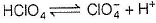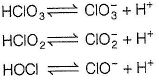Conjugate bases stabilises by resonance.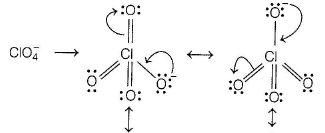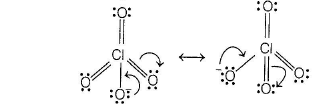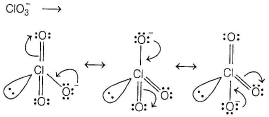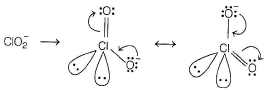CIO- does not show resonance. Thus, the stability order of conjugate due to response is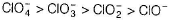Hence, the acidic strength of given oxoacids is as follows: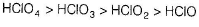QUESTION: 2

### Which one of the following properties is not Shown by NO? (JEE Main 2014)

Solution:

The electronic configuration of NO[15e-] is given by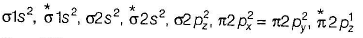Thus, NO possess one unpaired electron, hence it is paramagnetic in nature.  However, it dimerises at low temperatue to become diamagenetic.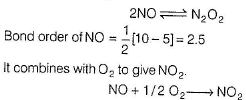*Multiple options can be correct
QUESTION: 3

### Which of the following is correct statement? (JEE Main 2013)

Solution:

(a) Number of electron in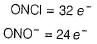Thus, ONCI and ONO- are not isoelectronic
(b) O3 has bent shape structure.
Central atom O is sp2-hybridised with one lone pair of electrons.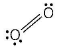(c) Ozone is violet black in solid state.
(d) O3 has no unpaired electrons, thus it is diamagnetic.
Hence, all the given statements are correct.

QUESTION: 4

Which of the following on thermal decomposition yields a basic as well as acidic oxide ?

(AIEEE 2012)

Solution: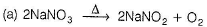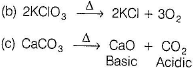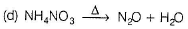QUESTION: 5

Which of the following statements is wrong ?

(AIEEE 2011)

Solution:

(a)Thermal stability of the hydrides decreases as we go down the group in periodic table for group 15 (N-family).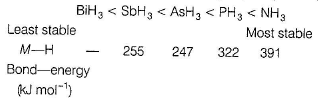(b) Due to absence ofd-orbital, nitrogen cannot formc/m-pm bond, thus it is correct.
(c) The N—N bond (BE 160 kJ mol-1) is weaker than P—P bond (BE 209 kJ mol -1). Thus, it is correct.
(d) N2O4 can form two resonance structures.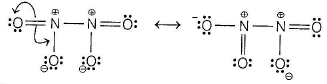Thus, it is correct.

QUESTION: 6

Which of the following statements regarding sulphur is in correct?

(AIEEE 2011)

Solution:

(a) S2 molecule is paramagnetic due to unpaired electrons in MO and is blue coloured compound, thus true.
(b) The vapour at 200°C consists mostly of S8 rings, thus correct.
(c) At 600°C, the gas mainly consists of S2 molecules, thus correct.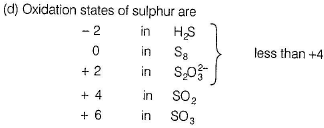QUESTION: 7

In which of the following arrangements, the sequence is not strictly according to the property written against it?

(AIEEE 2009)

Solution:

The correct increasing basic strength
SbH3 < AsH3 < PH3 < NH3
NH3 is most basic because of its small size, the electron density of electron pair is concentrated over small region.
As the size increases, the electron density gets diffused over a large surface area and hence, the ability to donate the electron pair (basicity) decreases. Hence, basic strength decreases down the group.

QUESTION: 8

Which one of the following reaction of xenon compounds is not feasible?

Solution:

XeF6 has much tendency to hydrolyse. The reverse reaction is more spontaneous.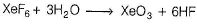QUESTION: 9

Regular use of which of the following fertilisers increases the acidity of soil?

(AIEEE 2007)

Solution: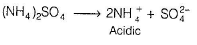QUESTION: 10

The number of hydrogen atom(s) attached to phosphorus atom in hypophosphorous acid is

(AIEEE 2005)

Solution:

Hypophosphorous acid (H3PO2) is a monobasic acid and has only one ionisable H because two H-atoms are directly attached to phosphorus.
The structure of H3PO2 is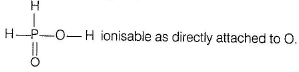QUESTION: 11

The correct order of the thermal stability of hydrogen halides (H—X) is

(AIEEE 2005)

Solution:

In a grop,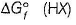changes from negative to positive downwaeds.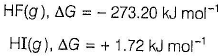Thus, HF is thermally stable and HI not.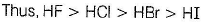QUESTION: 12

Which one of the following statements regarding helium is incorrect?

(AIEEE 2004)

Solution:

Helium is not used to produce and sustain powerful superconducting magnets. All others are the uses of helium.

QUESTION: 13

The substance used in Holmes signals of the ship is a mixture of

(AIEEE 2003)

Solution:

In Holmes signals of the ship, mixture of CaC2 and Ca3P2 is used.

QUESTION: 14

Concentrated hydrochloric acid when kept in open air, sometimes produces a cloud of white fumes. The explanation for it is that

(AIEEE 2003)

Solution: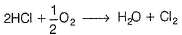QUESTION: 15

PCI3 and PCI5 both exist; NCl3 exists but NCI5 does not exist. It is due to

(AIEEE 2002)

Solution:

Phosphorus (3rd period element) can raise covalency facilitating vacant d-orbitals.
P (ground): [Ne] 3s2 3p3 3d0
P (excited): [Ne] 3s1 3p3 3d1